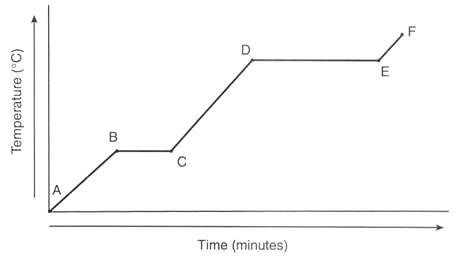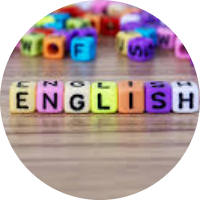# Entropy and Gibbs Free Energy

This quiz is based on the concepts in AP Chemistry Enduring understanding: "Chemical or physical processes are driven by a decrease in enthalpy or an increase in entropy or both." It also covers Enduring understanding: "The equilibrium constant is related to temperature and the difference in Gibbs free energy between reactants and products."

Start Quiz

Which of the following would most likely lead to a spontaneous reaction.

A low positive enthalpy.

A high negative enthalpy.

A high positive enthalpy.

A low negative enthalpy.

Your enthalpy is high and negative but your entropy is also negative. What type of temperature would you want to get a spontaneous reaction?

Low

High

Moderately high

Extremely high

Your enthalpy is +20 and your entropy is +5. Which temperature will give a spontaneous reaction?

4

0

8

10

A spontaneous reaction is more profitable to a company.

True

False

What line segment represents only the solid-state?A-B

B-C

D-E

C-D

A reaction, A⇆B, has a ΔH of -100 kJ/mol and a ΔS of -300 J/mol.K. Around what temperature will a 1:1 mixture of A and B be at equilibrium (i.e. ΔG = 0)?

0 degrees C

60 degrees C

120 degrees C

260 degrees C

What can be deduced about the ΔG of a reaction if the equilibrium constant K is greater than 1?

If K>1, then ΔG must be equal to K

If K>1, then ΔG must be negative

If K>1, then ΔG must be positive

There is no relationship between K and ΔG

A reaction has a ΔH of -100 kJ/mol and a ΔS of +100 J/mol.K. However, no reaction can be observed to be taking place. This is most likely because:

The enthalpy of the reaction is negative, preventing it from occurring.

The entropy of the reaction is positive, preventing it from occurring.

The entropy and enthalpy cancel each other out, preventing the reaction from occurring.

The reaction is thermodynamically favored but is prevented from occurring by a very high activation energy.

Quiz/Test Summary
Title: Entropy and Gibbs Free Energy
Questions: 8
Contributed by: